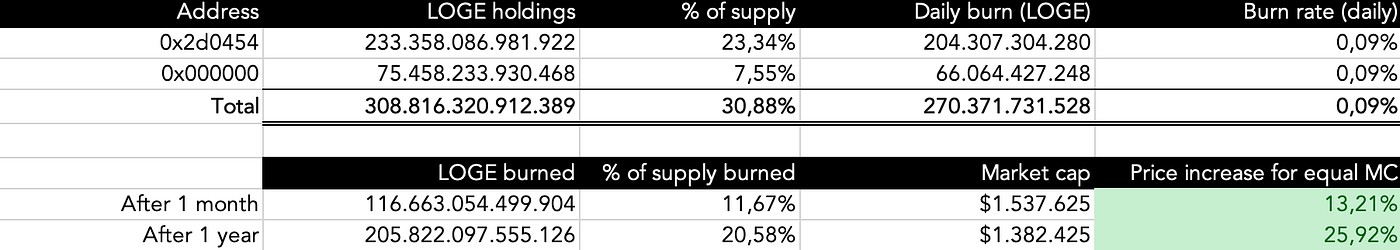# LunaDoge MATHS — PART 1

Welcome to the first LunaDoge mathematical analysis!

In this article, we will analyze the static rewards of LunaDoge. Based on our contract, each LOGE transaction is taxed 10%. Half of these taxes (5%) are proportionally distributed to holders based on LOGE held in your wallet. Often ignored or overlooked by a large portion of our community, understanding the maths behind the static rewards will propel LunaDoge to new ATHs 🚀. We highly encourage you to read this article, as understanding the maths behind LunaDoge will make you feel even more comfortable holding LOGE. You might even increase your stack by the time you’re finished with the article 👀.

Understanding LunaDoge static rewards

When it comes to understanding the rewards of LunaDoge, two variables are important. The first part is your LOGE held in terms of percentage of total supply. The second part is (daily) volume in \$.

Example 1: Anon holds 0.1% of the total supply (1,000,000,000,000 LOGE). The daily volume is \$100,000. The transaction taxes amount to \$10,000 for the day (10%). Of that \$10,000, half goes to static rewards. This means that \$5,000 will be distributed in LOGE to holders. Since Anon held 0.1% of the total supply, Anon is rewarded 0.1% times \$5,000 = \$5 for the day.

Example 2: Anon decided to become a LOGE whale and now holds 1% of the supply. Thanks to some quality shilling by Shill Team Six, LOGE reached a daily volume of \$1,000,000. Thanks to the increased volume, the daily rewards have increased to \$50,000. Because Anon is a LOGE whale, he now receives 1% times \$50,000 = \$500 for the day.

Based on the examples above, you can see that rewards increase with an increase in volume, holdings or both. When you take price into account, a lower price leads to a higher amount of LOGE given equal volume. Similarly, a higher price will lead to fewer LOGE in rewards given equal volume.

Introduction

Before we calculate the possible scenarios, let’s take a look at the current statistics of LunaDoge. When we started writing this article, the LOGE price was \$0,00000000174, the market cap was \$1,740,700 and the amount of LOGE burned was 43.9 trillion (4.39% of total supply). We will use these statistics in our analyses.

Furthermore, we will add the upcoming buyback and burn to our scenario. Based on the current LP holdings a total of 64.9 trillion LOGE (6.49% of total supply) will be burned, resulting in a total amount of 108.8 trillion LOGE burned (10.88% of total supply). To top it all off, the team tokens (200 trillion LOGE) are locked in a contract for 3 to 6 months. The rewards accrued by the contract are considered a burn as well, since a contract is unable to move a token to another address. In summary, 308.8 trillion LOGE (30.88% of total supply) will burn their LOGE rewards. When we take example 2 as our scenario, 30.88% of the \$50,000 daily rewards (\$15,440) will be burned. Since these addresses are unable to move their tokens, the burn share of the total supply will increase perpetually 🔥.Analysis 1: \$30,480 Average Daily Volume (“ADV”) — No price change

Using CoinGecko historical data, the current ADV for LOGE is \$30,480. Daily rewards amount to 5% times \$30,480 = \$1,524. Since 30.88% of the total supply will burn their rewards, \$1,524 times 30.88% = \$470.64 in LOGE will be burned. Given the current LOGE price of \$0,00000000174, 270,371,731,528 LOGE will be added to the burn addresses on day 1, resulting in a higher burn share of the total supply for the next day.

Extrapolating the data leads to a total burn of 116.7 trillion LOGE (11.67% of total supply) after 1 month and 205.8 trillion LOGE after 1 year (20.58% of total supply). Because the circulating supply decreases with each token burned, price needs to increase to keep an equal market cap. This is the most basic analysis, not taking price swings into account and assuming a steady low volume.

The current ATH ADV for LOGE according to CoinGecko is \$423,777. If we use this ADV, daily static rewards are 5% times \$423,777 = \$21,189. This results in 30.88% times \$21,189 = \$6,543 in LOGE being burned. Given the current LOGE price of \$0,00000000174, 3,759,071,855,527 LOGE will be added to the burn addresses on day 1. LOGE burned after 1 month and 1 year increased immensely compared to our basic scenario. 21.75% of total supply will be burned in 1 month and 64.68% of total supply will be burned in 1 year.

Because price usually reacts to scarcity, in this scenario we will have the price react to the token burn. A \$30,480 ADV results in a burn rate of 0.087551%. If we want the price to react to keep up with the decreasing supply, it needs to increase by (1 — (1 + 0.087551%)) = 0.87628%. Price will increase by 2.57% after the first month and 37.51% after one year.

Example 3: Anon has diamond hands and held his whale stack for one year. Anon paid \$1,740 for his stack of 1,000,000,000,000 LOGE. Thanks to the static rewards, the 1,000,000,000,000 LOGE have increased to 1,313,494,916,809 LOGE (+31.35%). Because the price increased by 37.51% as well, Anon now sits on a stack of LOGE worth \$3,144 (+80.62% compared to his buy-in).